# NCERT Solutions for Class 6 Maths Chapter 8: Decimals Deleted Exercises

In this page we have NCERT Solutions for Class 6 Maths Chapter 8: Decimals Deleted Exercises as per rationalized syllabus. Hope you like them and do not forget to like , social share and comment at the end of the page.

## Exercise 8.1

Question 1

Write the following as numbers in the given table.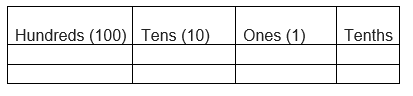One block divided into 10 equal parts means each part is 1/10 (one-tenth) of a unit. It can be written as 0.1 in decimal notation. One block divided into 100 equal parts means each part is (1/100) one-hundredth of a unit.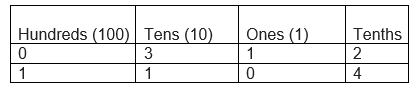Question 2
Write the following decimals in the place value table.
(a) 19.4
(b) 0.3
(c) 10.6
(d) 205.9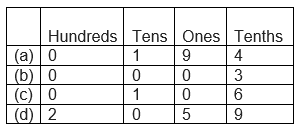Question 3
Write each of the following as decimals:
(a) Seven-tenths
(b) Two tens and nine-tenths
(c) Fourteen-point six
(d) One hundred and two ones
(e) Six-hundred-point eight

(a)Seven-tenths

=7/10 =.7
(b) Two tens and nine tenths = 20 + 9/10 =20 +.9=20.9
(c)Fourteen point six = 14.6
(d) One hundred and two ones = 100 + 2 = 102
(e) Six hundred point eight = 600.8

Question 4
Write each of the following as decimals:
(a) $\frac {5}{10}$
(b) $3 + \frac {7}{10}$
(c) $200 + 60 +5 + \frac {1}{10}$
(d) $70+ \frac {8}{10}$
(e) $\frac {88}{10}$
(f) $4 \frac {2}{10}$
(g) $\frac {3}{2}$ (h) $\frac {2}{5}$
(i) $\frac {12}{5}$
(j)$3 \frac {3}{5}$
(k)$4 \frac {1}{2}$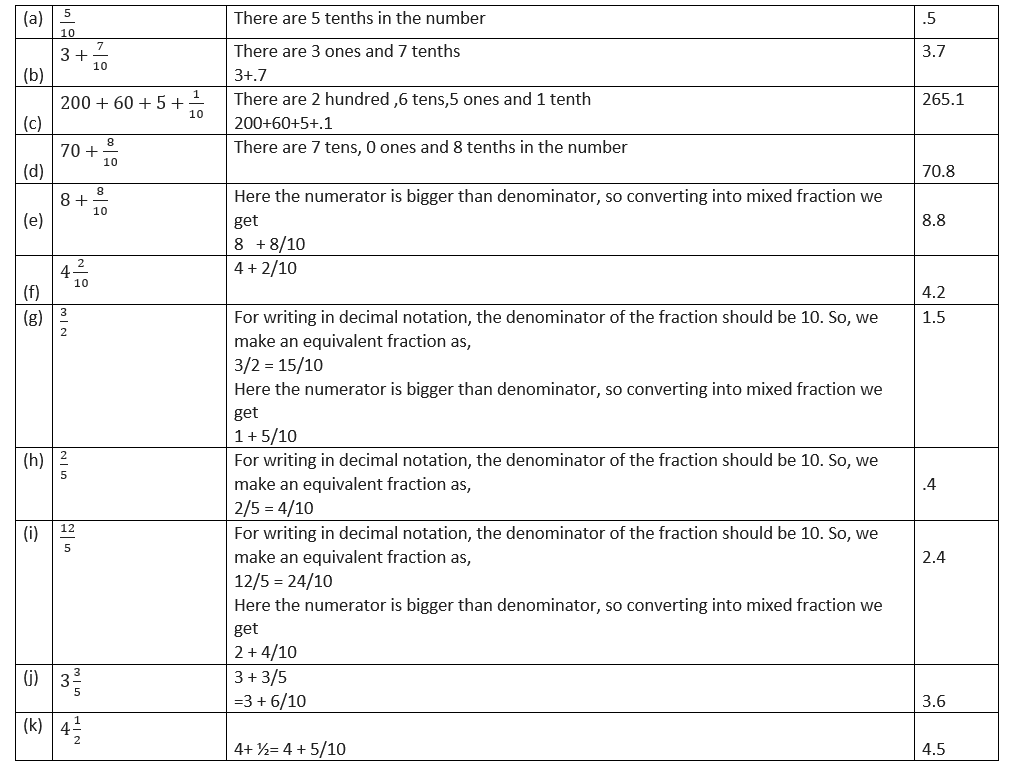Question 5
Write the following decimals as fractions. Reduce the fractions to lowest form.
(a) 0.6
(b) 2.5
(c) 1.0
(d) 3.8
(e) 13.7
(f) 21.2
(g) 6.4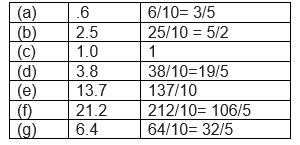Question 6
Express the following as cm using decimals.
(a) 2 mm
(b) 30 mm
(c) 116 mm
(d) 4 cm 2 mm
(e) 162 mm
(f) 83 mm
We know that 10 mm =1 cm i.e.   1 mm is 1/10 of centimeter
(a)2 mm = 2/10 cm = .2 cm
(b) 30mm = 30/10 cm = 3 cm
(c) 116 mm= 116/10 cm = 11.6 cm
(d) 4 cm 2 mm= (4 + 2/10) cm = 4.2 cm
(e) 162 mm = 162/10 cm = 16.2 cm
(f) 83 mm = 83/10 cm = 8.3 cm

Question 7
Between which two whole numbers on the number line are the given numbers lie? Which of these whole numbers is nearer the number?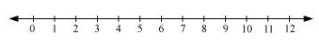(a) 0.8
(b) 5.1
(c) 2.6
(d) 6.4
(e) 9.1
(f) 4.9

(a) 0.8 = 0 + 8/10

So, it lies between 0 and 1 and is nearer to 1.
(b)5.1 = 5 + 1/10

So, it lies between 5 and 6 and is nearer to 5.
(c)2.6 = 2 + 6/10

So, it lies between 2 and 3 and is nearer to 3.
(d) 6.4 = 6 + 4/10

So, it lies between 6 and 7 and is nearer to 6.
(e) 9.1 = 9 + 1/10

So, it lies between 9 and 10 and is nearer to 9.
(f) 4.9 = 4 + 9/10

So, it lies between 4 and 5 and is nearer to 5.

Question 8
Show the following numbers on the number line.

(a) 0.2

(b) 1.9

(c) 1.1

(d) 2.5

a)      0.2 lies between 0 and 1. There are 2 tenths in it. Divide the unit length into 10 equal parts and 0.2 represents the 2nd part.

b)      1.9 lies between 1 and 2. There are 9 tenths in it. 1.9 represents the ninth part after 1.

c)      1.1 lies between 1 and 2. There are 1 tenth in it. 1.1 represents the first part after 1.

d)     2.5 lies between 2 and 3. There are 5 tenths in it. 2.5 represents the fifth part after 2.

Question 9

Write the decimal number represented by the points A, B, C, D on the given number line?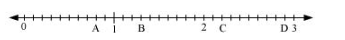Each interval is divided in ten equal parts and we can just count the part to get the value
 a) A A represents the 8th equal part between 0 and 1. So, A is 0.8. b) B B represents the 3rd equal part between 1 and 2. So, B is 1.3 c) C C represents the 2nd equal part between 2 and 3 and hence is the number 2.2 d) D D is 2.9, the 9th equal part between 2 and 3.

Question 10

(a) The length of Ramesh’s notebook is 9 cm 5 mm. What will be its length in cm?

(b) The length of a young gram plant is 65 mm. Express its length in cm.

We know that 10 mm =1 cm i.e.   1 mm is 1/10 of centimeter

a) length= 9cm5mm = 9 + 5/10= 9.5 cm

b) length= 65mm =65/10= 6.5 cm

## Exercise 8.2

Question 1
Complete the table with the help of these boxes and use decimals to write the number.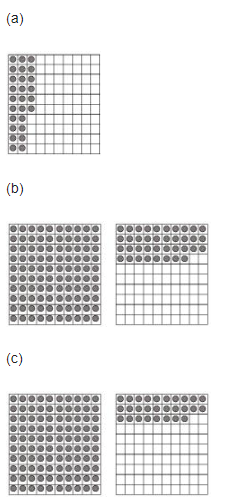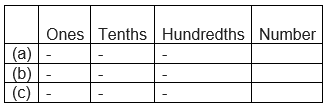First counting the boxes in each figure we get
(a) There are two tenths and 6 hundredths. So, the decimal is 0.26
(b) One whole or ones, 3 tenths and 8 hundredths. Decimal is 1.38
(c) One whole or ones, two tenths and 8 hundredths. Decimal is 1.28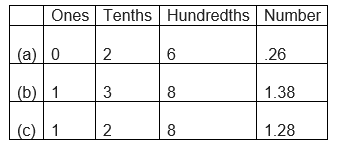Question 2

Write the numbers given in the following place value table in decimal form.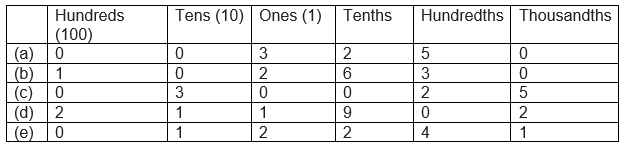a) $0+0+3+\frac {2}{10}+\frac {5}{100}+ \frac {0}{1000}=3+ .2+ .05=3.25$ b) $100+0+2+ \frac {6}{10} + \frac {3}{100} + \frac {0}{1000}= 100+2+.6+.03= 102.63$ c) $0+30+0+0+ \frac {2}{100} + \frac {5}{1000}=30+ +.02+.005=30.025$ d) $200+ 10+1+ \frac {9}{10} + \frac {0}{100} + \frac {2}{10000}= 211+.9+.002=211.902$ e) $0+ 10+2+ \frac {2}{10} + \frac {4}{100} + \frac {1}{1000}= 12+.2+.04+.001= 12.241$

Question 3

Write the following decimals in the place value table.

(a) 0.29

(b) 2.08

(c) 19.60

(d) 148.32

(e) 200.812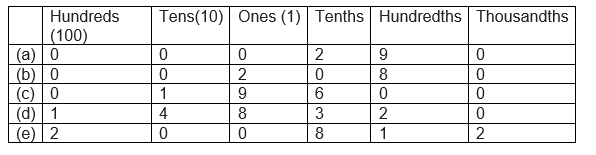Question 4

Write each of the following decimals.

(a)$20 + 9 + \frac {4}{10} + \frac {1}{100}$

(b)$137 + \frac {5}{100}$

(c)$\frac {7}{10} + \frac {6}{100} + \frac {4}{1000}$

(d)$23 + \frac {2}{10} + \frac {6}{1000}$

(e)$700 + 20 + 5 + \frac {9}{100}$

 a) $20 + 9 + \frac {4}{10} + \frac {1}{100}$ 29+.4+.01=29.41 b) $137 + \frac {5}{100}$ 137+.05= 137.05 c) $\frac {7}{10} + \frac {6}{100} + \frac {4}{1000}$ .7+.06+.004=.764 d) $23 + \frac {2}{10} + \frac {6}{1000}$ 23+.2+.006=23.206 e) $700 + 20 + 5 + \frac {9}{100}$ 725+.09=725.09

Question 5

Write each of the following decimals in words.

(a) 0.03

(b) 1.20

(c) 108.56

(d) 10.07

(e) 0.032

(f) 5.008

a)      0.03 = zero point zero three

b)      1.20 = one point two zero

c)      108.56 = one hundred eight point five six.

d)     10.07 = ten point zero seven.

e)      0.032 = zero point zero three two

f)       5.008 = five point zero zero eight

Question 6

Between which two numbers in tenths place on the number line does each of the given number lie?

(a) 0.06

(b) 0.45

(c) 0.19

(d) 0.66

(e) 0.92

(f) 0.57

1.      0.06 lies between 0 and 0.1

2.      0.45 lies between 0.4 and 0.5

3.      0.19 lies between 0.1 and 0.2

4.      0.66 lies between 0.6 and 0.7

5.      0.92 lies between 0.9 and 1

6.      0.57 lies between 0.5 and 0.6

Question 7

Write as fractions in lowest terms.

(a) 0.60

(b) 0.05

(c) 0.75

(d) 0.18

(e) 0.25

(f) 0.125

(g) 0.066

a) .60= 60/100=3/5
b)  .05= 5/100=1/20
c) .75= 75/100= 3/4
d) .18= 18/100=9/50
e) .25= 25/100=1/4
f) .125= 125/1000=1/8
g) .066= 66/1000=      33/500### Practice Question

Question 1 What is $\frac {1}{2} + \frac {3}{4}$ ?
A)$\frac {5}{4}$
B)$\frac {1}{4}$
C)$1$
D)$\frac {4}{5}$
Question 2 Pinhole camera produces an ?
A)An erect and small image
B)an Inverted and small image
C)An inverted and enlarged image
D)None of the above